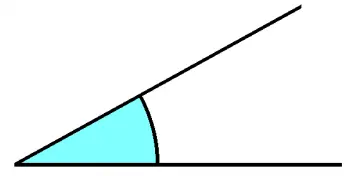# Convex Angle: Characteristics, Definition and ExamplesThe convex angle is a type of angle that is defined based on its measure. According to the classification of angles by measure, the convex angle measures less than 180º or π. Therefore, its dimension lies between a zero angle and a flat angle.

An angle is formed by two lines, segments, or rays intersecting at the vertex. The angle measure determines the inclination of one segment to the other.

## Convex Angle Characteristics

• The difference between a convex angle and a concave angle is that the former is always less than 180, and the latter is always greater than 180.

• The remaining angle formed by the exterior angle of the convex angle that is not a straight angle is a concave angle, which measures between 180º and 360º. The sum of both angles forms a complete angle of 360º.

• All angles of a convex polygon are convex since they are less than 180.

## Classification of Convex Angles

Depending on the magnitude, the three types of convex angles that divide them are:

• Acute convex angle: The acute angle has a dimension less than 90º.

• Obtuse convex angle: Obtuse angles measure between 90º and 180º.

• Right convex angle: The right angle is that angle whose size is 90º.

## What Is a Convex Polygon?

A convex polygon is a polygon whose angles are less than 180 degrees which is the case of all regular (convex) polygons. On the contrary, we define a concave polygon if at least one of its interior angles is greater than 180.

An irregular convex polygon is a polygon with all its angles are convex but with different dimensions.

The sum of the interior angles of a polygon is the same as the number of sides and vertices of the polygon.

## Concave Vs. Convex Polygon

The differences between convex and concave polygons are the following:

• All vertices of a convex polygon point outwards. On the other side, at least one vertex point inwards in a concave polygon.

• At least one interior angle of a concave polygon is greater than 180: Meanwhile, all angles of a convex polygon are less than 180.

• All lines joining two vertices of a convex polygon lie entirely in it.

## Convex Angle Examples

Let's see some examples of convex angles:

• All interior angles of a square. As we have explained, all regular polygons are formed by angles less than 180º.

• The tip of an arrow forms a convex angle.

• The angle formed by the letter “V” is less than 180.

• The angle formed when we separate the fingers of the hands.

Author:

Published: February 28, 2023
Last review: February 28, 2023